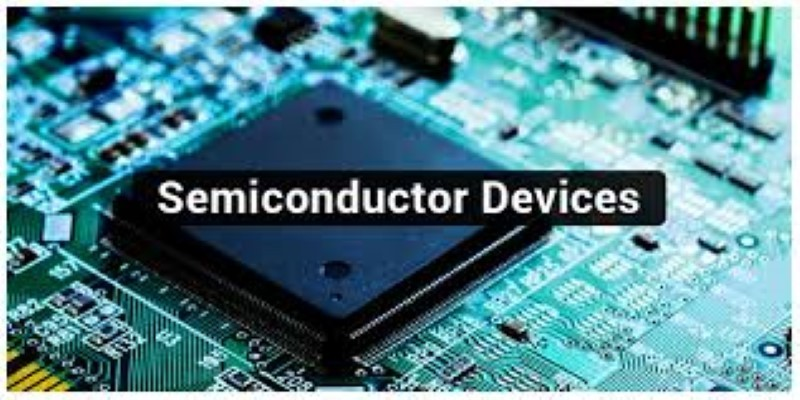0(0)

# Fundamentals of semiconductor devices

• Course level: All Levels

#### This course provides the essential foundations required to understand the operation of semiconductor devices such as transistors, diodes, solar cells, light-emitting devices, and more. The material will primarily appeal to electrical engineering students whose interests are in applications of semiconductor devices in circuits and systems. The treatment is physical and intuitive, and not heavily mathematical.

Technology users will gain an understanding of the semiconductor physics that is the basis for devices. Semiconductor technology developers may find it a useful starting point for diving deeper into condensed matter physics, statistical mechanics, thermodynamics, and materials science. The course presents an electrical engineering perspective on semiconductors, but those in other fields may find it a useful introduction to the approach that has guided the development of semiconductor technology for the past 50+ years.

### Who can take this course?

-Engineering Students

#### What Will I Learn?

• Students will learn about the following specific topics:
• energy bands
• band gaps
• effective masses
• electrons and holes
• basics of quantum mechanics
• the Fermi function
• the density-of-states
• intrinsic carrier density
• doping and carrier concentrations
• carrier transport
• generation-recombination
• quasi-Fermi levels
• the semiconductor equations
• energy band diagrams

59 Lessons

#### Fundamentals of semiconductor devices

##### mod12lec5900:00:0035 £